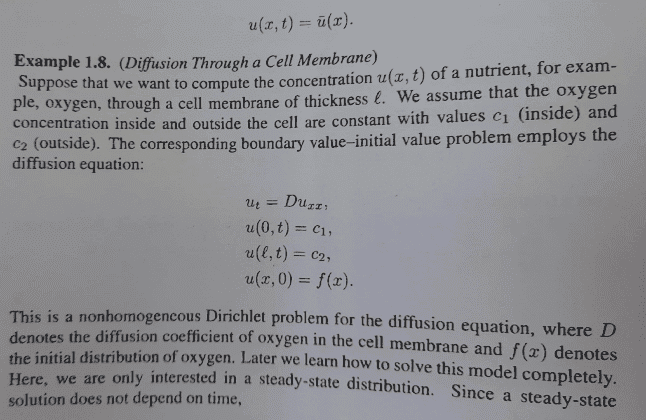# Determining whether the Dirichlet problem is nonhomogenous

• A
• chwala

#### chwala

Gold Member
TL;DR Summary
See attachedI am going through the literature on Dirichlet and Neumann conditions for the heat equation...i need to be certain on the nonhomogenous bit that is indicated on the excerpt. I am aware that for a pde to be considered homogenous, ##Lu=0## and for it to be non homogenous; ##Lu=f##, where ##L## is the given differential operator.

To determine whether the pde is homogenous or not we make use of the boundary conditions, correct?

In our case we have the given pde with two boundary conditions and one initial condition (not considered due to steady-state condition).

I now get it!
thus to find the solution, we note that since the steady-state is not dependant on time, then it follows that, ##u_{t}=0##
##u(x,t)=u(x)## ##Du_{xx}## =##0→u_{xx}=0## on integration we shall have
##u_{x}=M##, where ##M## is a constant. Integrating again yields
##u(x)=Mx+N##, where ##N## is also a constant. Now using the first boundary conditions, ##u(0,t)=c_1##, we shall have
## c_1=N## on using the second boundary condition, we shall have
##c_2=Ml+c_1## it follows that ##u(x)##=##\dfrac {c_2-c_1}{l}##+##c_1##
cheers guys...

#### Attachments

Last edited:

Summary: See attached

View attachment 300372
I am going through the literature on Dirichlet and Neumann conditions for the heat equation...i need to be certain on the nonhomogenous bit that is indicated on the excerpt. I am aware that for a pde to be considered homogenous, ##Lu=0## and for it to be non homogenous; ##Lu=f##, where ##L## is the given differential operator.

To determine whether the pde is homogenous or not we make use of the boundary conditions, correct?

There are two ways in which a PDE can be non-homogenous.

(1): The right hand side can be non-zero, as in $L(u) = f$.

(2) A boundary condition can be non-homogenous, ie. of the form $$\alpha u + \beta \frac{\partial u}{\partial n} = g$$ where $\alpha$ and $\beta$ are functions of position and time satisfying $\alpha^2 + \beta^2 = 1$.

In our case we have the given pde with two boundary conditions and one initial condition (not considered due to steady-state condition).

I now get it!
thus to find the solution, we note that since the steady-state is not dependant on time, then it follows that, ##u_{t}=0##
##u(x,t)=u(x)## ##Du_{xx}## =##0→u_{xx}=0## on integration we shall have
##u_{x}=M##, where ##M## is a constant. Integrating again yields
##u(x)=Mx+N##, where ##N## is also a constant. Now using the first boundary conditions, ##u(0,t)=c_1##, we shall have
## c_1=N## on using the second boundary condition, we shall have
##c_2=Ml+c_1## it follows that ##u(x)##=##\dfrac {c_2-c_1}{l}##+##c_1##
cheers guys...

Your final expression for $u(x)$ does not contain $x$. It is easiest to set $$\begin{split} u(x) &= c_2\frac xl + c_1\left(1 - \frac xl\right) + v(x) \\ &= c_1 + (c_2 - c_1)\frac xl + v(x) \end{split}$$ where $v$ satisfies $$v_{xx} = -(c_1 + (c_2-c_1)x/l)_{xx} = 0$$ subject to the homogenous condition $v(0) = v(l) = 0$. This forces $v(x) = 0$.

•chwala
TL;DR Summary: See attached

View attachment 300372
I am going through the literature on Dirichlet and Neumann conditions for the heat equation...i need to be certain on the nonhomogenous bit that is indicated on the excerpt. I am aware that for a pde to be considered homogenous, ##Lu=0## and for it to be non homogenous; ##Lu=f##, where ##L## is the given differential operator.

To determine whether the pde is homogenous or not we make use of the boundary conditions, correct?

In our case we have the given pde with two boundary conditions and one initial condition (not considered due to steady-state condition).

I now get it!
thus to find the solution, we note that since the steady-state is not dependant on time, then it follows that, ##u_{t}=0##
##u(x,t)=u(x)## ##Du_{xx}## =##0→u_{xx}=0## on integration we shall have
##u_{x}=M##, where ##M## is a constant. Integrating again yields
##u(x)=Mx+N##, where ##N## is also a constant. Now using the first boundary conditions, ##u(0,t)=c_1##, we shall have
## c_1=N## on using the second boundary condition, we shall have
##c_2=Ml+c_1## it follows that ##u(x)##=##\dfrac {c_2-c_1}{l}##+##c_1##
cheers guys...
Amended...

it follows that ##u(x)##=##\dfrac {c_2-c_1}{l}x##+##c_1##